Ex 9.4

Chapter 9 Class 12 Differential Equations
Serial order wise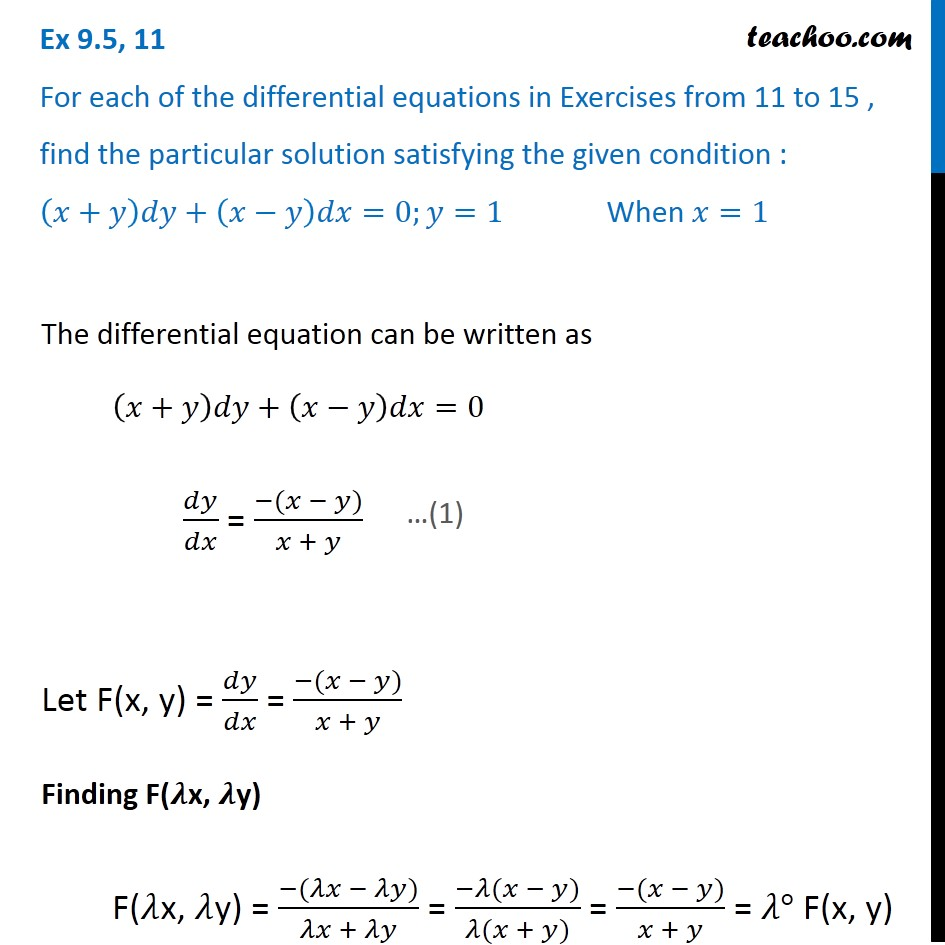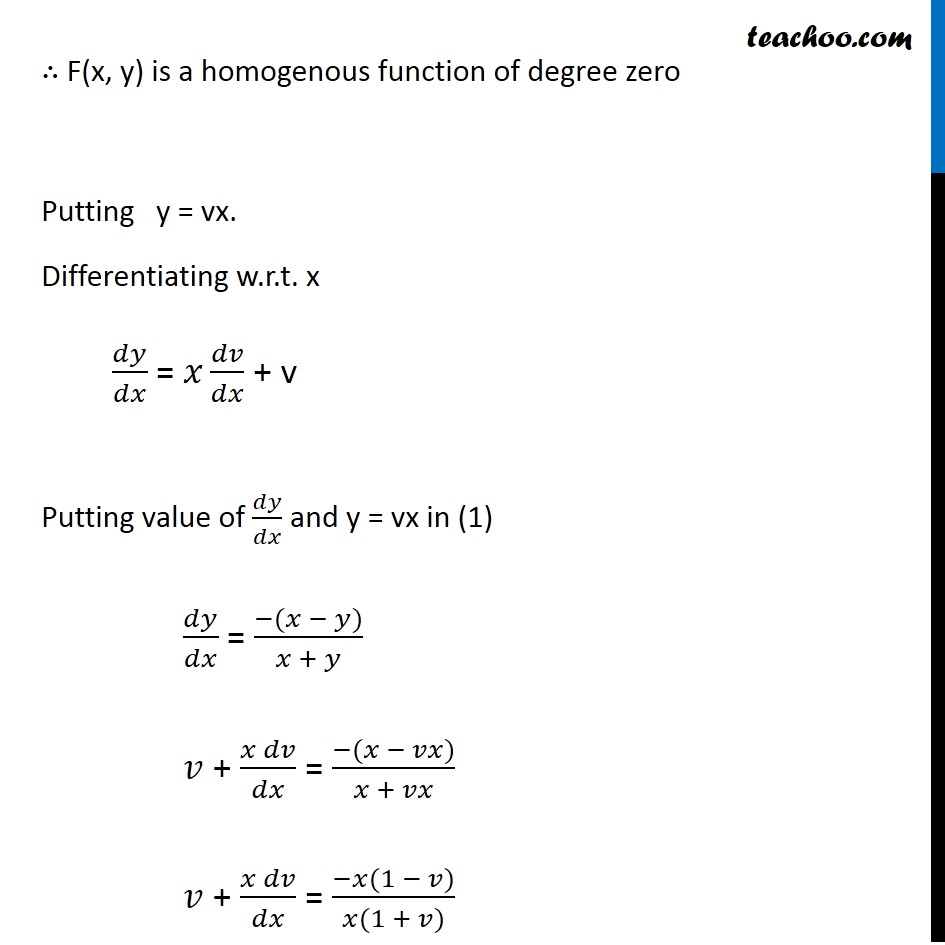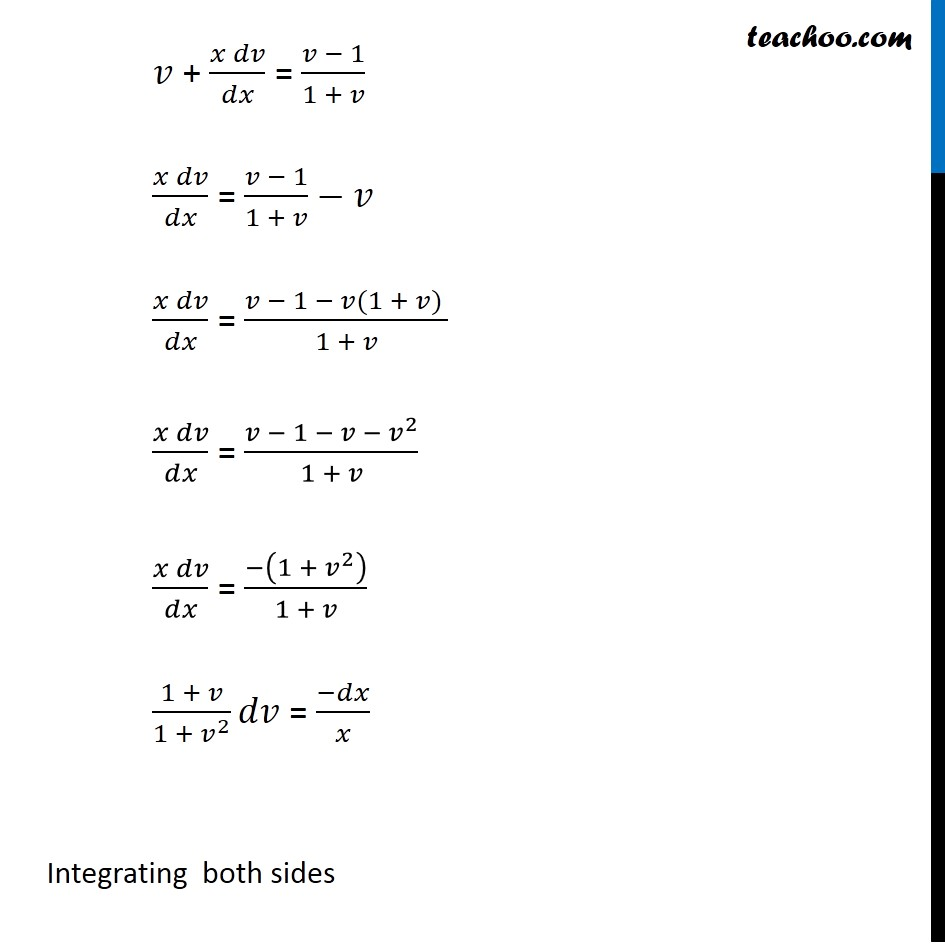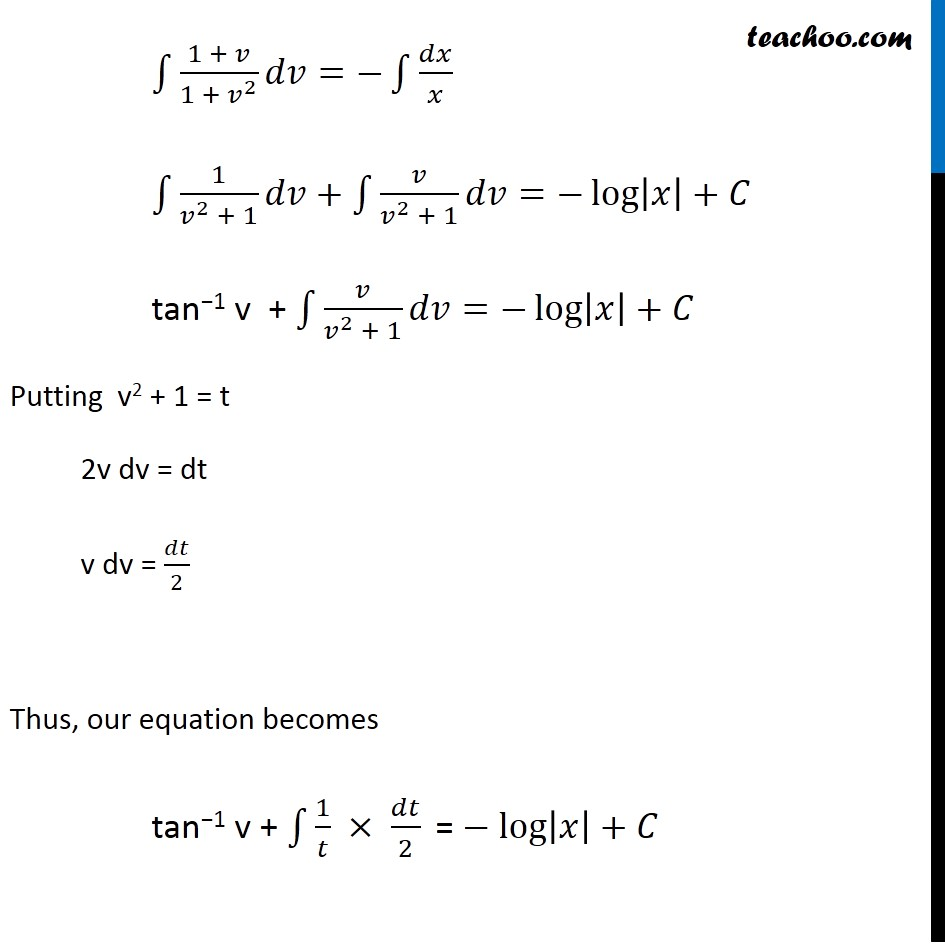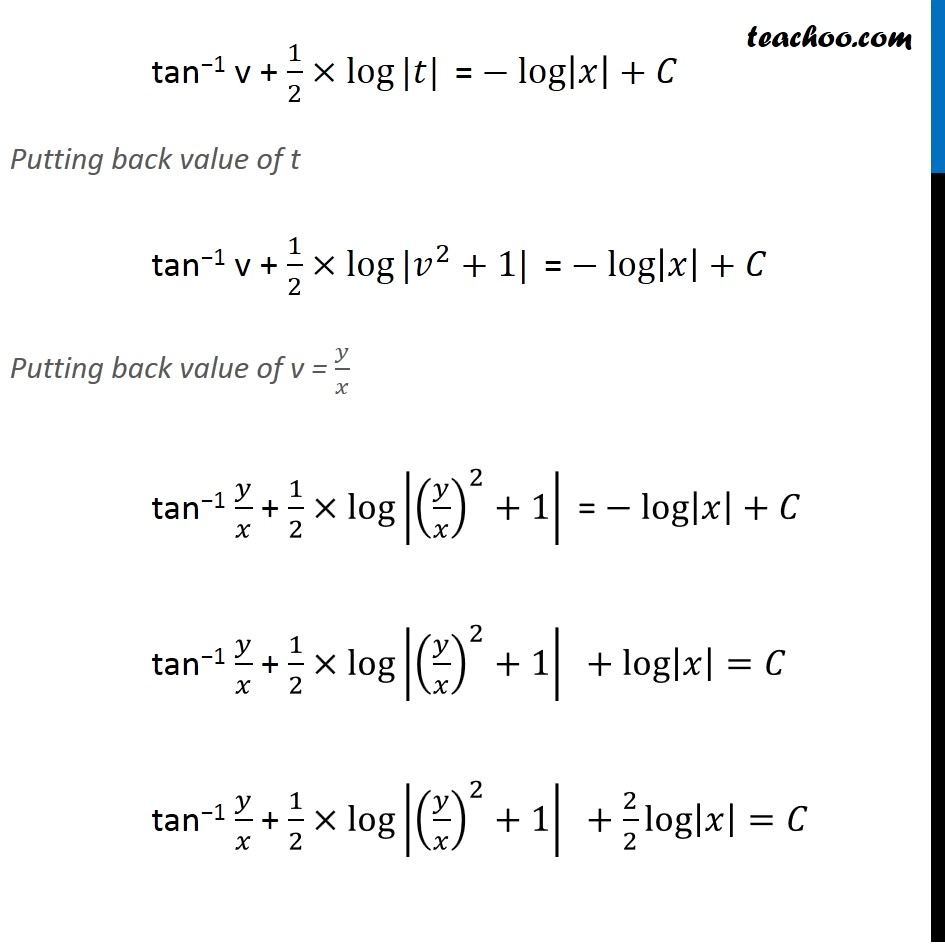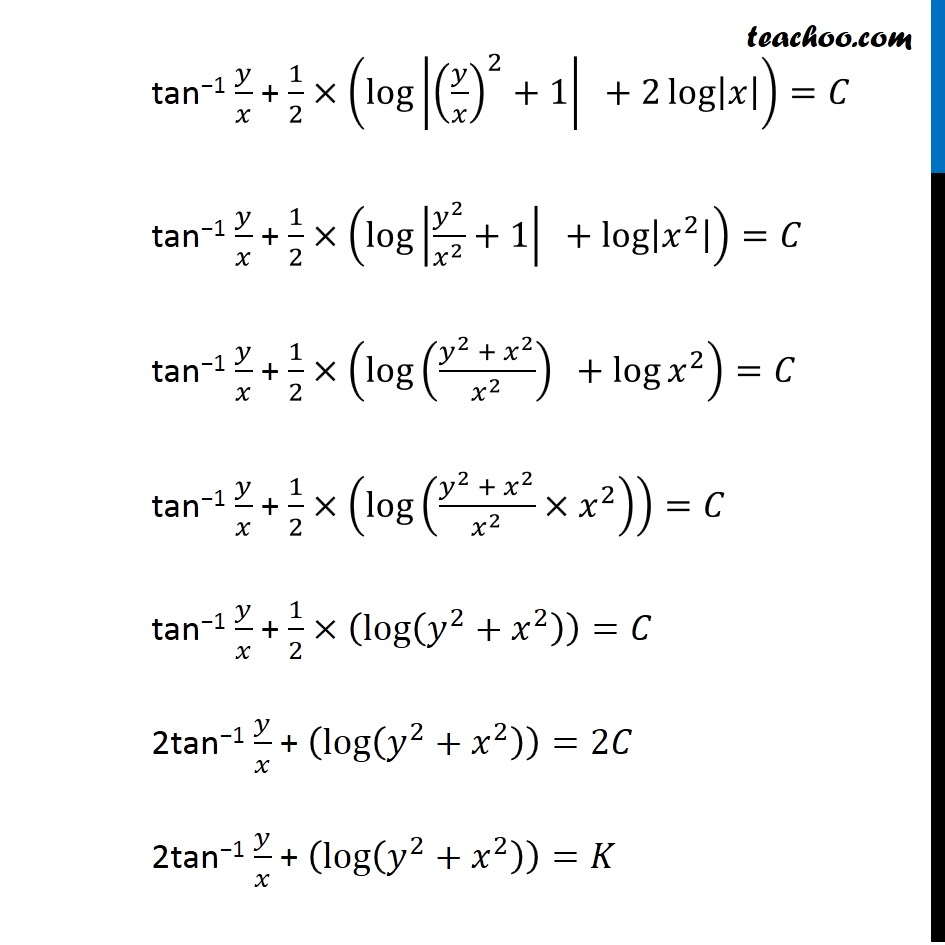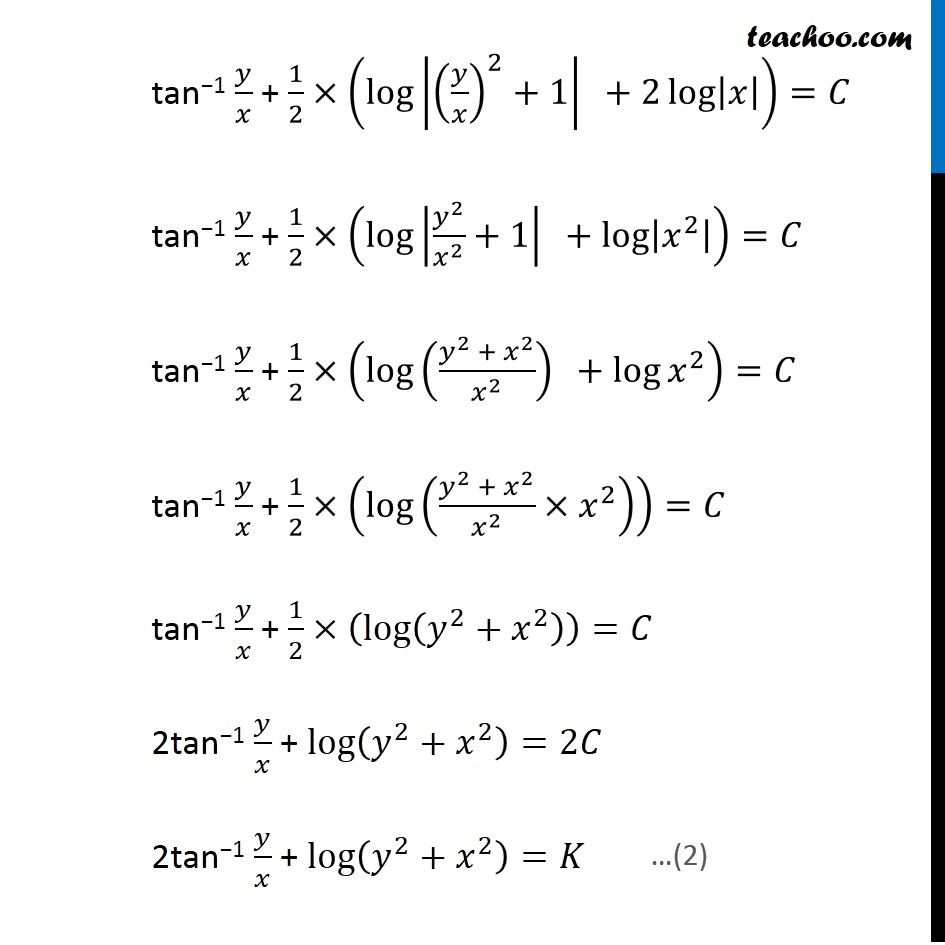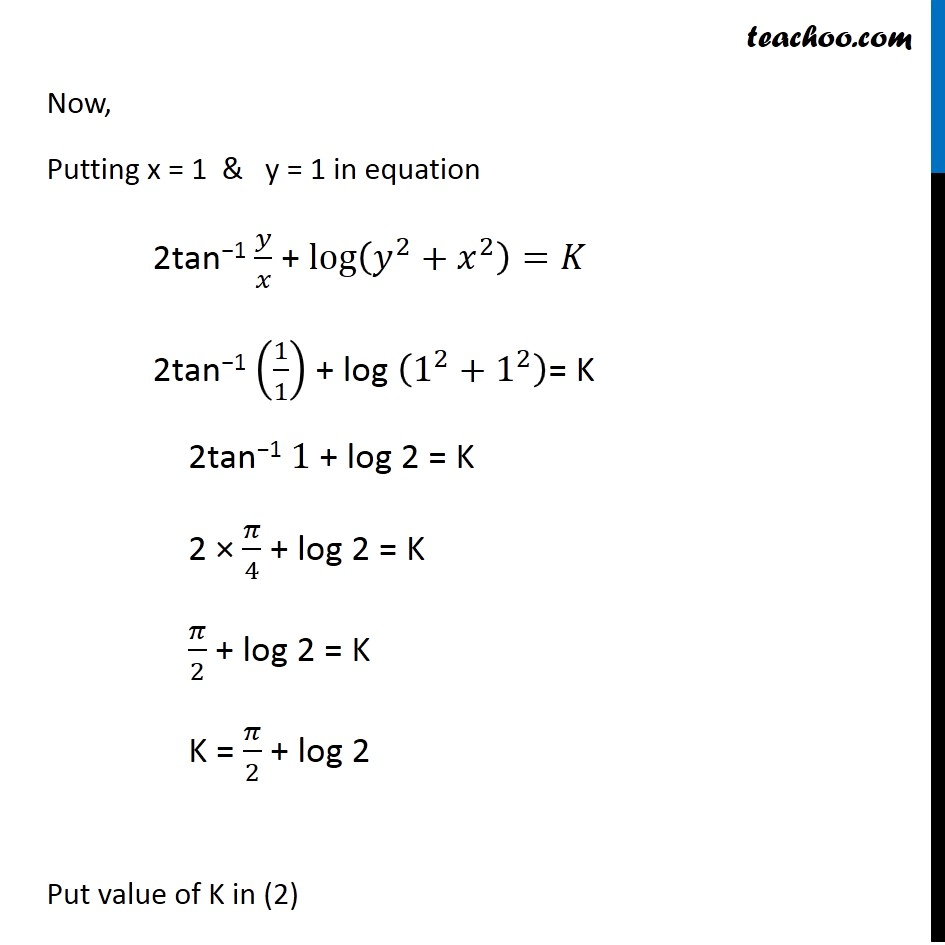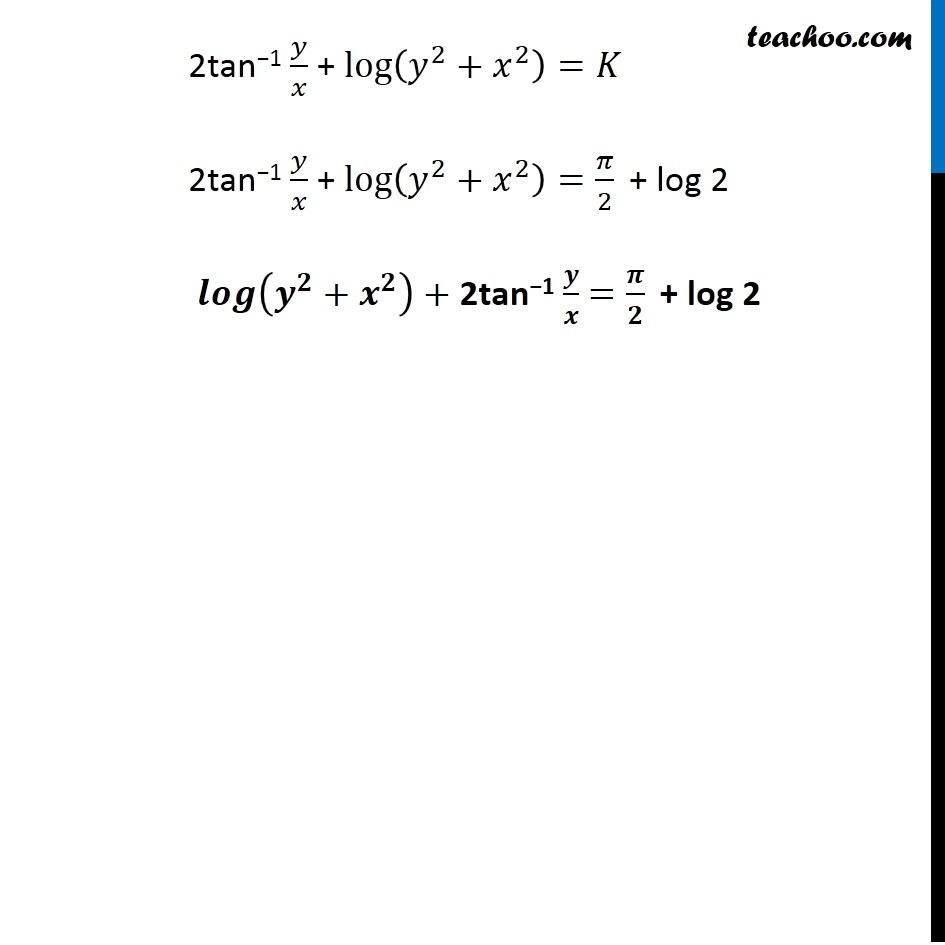Learn in your speed, with individual attention - Teachoo Maths 1-on-1 Class

### Transcript

Ex 9.4, 11 For each of the differential equations in Exercises from 11 to 15 , find the particular solution satisfying the given condition : (𝑥+𝑦)𝑑𝑦+(𝑥−𝑦)𝑑𝑥=0;𝑦=1 When 𝑥=1 The differential equation can be written as (𝑥+𝑦)𝑑𝑦+(𝑥−𝑦)𝑑𝑥=0 𝑑𝑦/𝑑𝑥 = (−(𝑥 − 𝑦))/(𝑥 + 𝑦) Let F(x, y) = 𝑑𝑦/𝑑𝑥 = (−(𝑥 − 𝑦))/(𝑥 + 𝑦) Finding F(𝝀x, 𝝀y) F(𝜆x, 𝜆y) = (−(𝜆𝑥 − 𝜆𝑦))/(𝜆𝑥 + 𝜆𝑦) = (−𝜆(𝑥 − 𝑦))/(𝜆(𝑥 + 𝑦)) = (−(𝑥 − 𝑦))/(𝑥 + 𝑦) = 𝜆° F(x, y) ∴ F(x, y) is a homogenous function of degree zero Putting y = vx. Differentiating w.r.t. x 𝑑𝑦/𝑑𝑥 = 𝑥 𝑑𝑣/𝑑𝑥 + v Putting value of 𝑑𝑦/𝑑𝑥 and y = vx in (1) 𝑑𝑦/𝑑𝑥 = (−(𝑥 − 𝑦))/(𝑥 + 𝑦) 𝑣 + (𝑥 𝑑𝑣)/𝑑𝑥 = (−(𝑥 − 𝑣𝑥))/(𝑥 + 𝑣𝑥) 𝑣 + (𝑥 𝑑𝑣)/𝑑𝑥 = (−𝑥(1 − 𝑣))/(𝑥(1 + 𝑣)) 𝑣 + (𝑥 𝑑𝑣)/𝑑𝑥 = (𝑣 − 1)/(1 + 𝑣) (𝑥 𝑑𝑣)/𝑑𝑥 = (𝑣 − 1)/(1 + 𝑣)−𝑣 (𝑥 𝑑𝑣)/𝑑𝑥 = (𝑣 − 1 − 𝑣(1 + 𝑣) )/(1 + 𝑣) (𝑥 𝑑𝑣)/𝑑𝑥 = (𝑣 − 1 − 𝑣 − 𝑣^2)/(1 + 𝑣) (𝑥 𝑑𝑣)/𝑑𝑥 = (−(1 + 𝑣^2 ))/(1 + 𝑣) (1 + 𝑣)/(1 + 𝑣^2 ) 𝑑𝑣 = (−𝑑𝑥)/𝑥 Integrating both sides ∫1▒〖(1 + 𝑣)/(1 + 𝑣^2 ) 𝑑𝑣=−∫1▒𝑑𝑥/𝑥〗 ∫1▒〖1/(𝑣^2 + 1) 𝑑𝑣+∫1▒𝑣/(𝑣^2 + 1) 𝑑𝑣=−log⁡|𝑥|+𝐶〗 ∫1▒〖(1 + 𝑣)/(1 + 𝑣^2 ) 𝑑𝑣=−∫1▒𝑑𝑥/𝑥〗 ∫1▒〖1/(𝑣^2 + 1) 𝑑𝑣+∫1▒𝑣/(𝑣^2 + 1) 𝑑𝑣=−log⁡|𝑥|+𝐶〗 tan−1 v + ∫1▒𝑣/(𝑣^2 + 1) 𝑑𝑣=−log⁡|𝑥|+𝐶 Putting v2 + 1 = t 2v dv = dt v dv = 𝑑𝑡/2 Thus, our equation becomes tan−1 v + ∫1▒〖1/𝑡 × 𝑑𝑡/2 " =" −log⁡|𝑥|+𝐶" " 〗 tan−1 v + 1/2×log⁡〖|𝑡|〗 " ="−log⁡|𝑥|+𝐶 Putting back value of t tan−1 v + 1/2×log⁡〖|𝑣^2+1|〗 " ="−log⁡|𝑥|+𝐶 Putting back value of v = 𝑦/𝑥 tan−1 𝑦/𝑥 + 1/2×log⁡|(𝑦/𝑥)^2+1| " ="−log⁡|𝑥|+𝐶 tan−1 𝑦/𝑥 + 1/2×log⁡|(𝑦/𝑥)^2+1| " "+log⁡|𝑥|=𝐶 tan−1 𝑦/𝑥 + 1/2×log⁡|(𝑦/𝑥)^2+1| " "+2/2 log⁡|𝑥|=𝐶 tan−1 𝑦/𝑥 + 1/2×(log⁡|(𝑦/𝑥)^2+1| " " +2 log⁡|𝑥| )=𝐶 tan−1 𝑦/𝑥 + 1/2×(log⁡|𝑦^2/𝑥^2 +1| " " +log⁡|𝑥^2 | )=𝐶 tan−1 𝑦/𝑥 + 1/2×(log⁡((𝑦^2 + 𝑥^2)/𝑥^2 )" " +log⁡〖𝑥^2 〗 )=𝐶 tan−1 𝑦/𝑥 + 1/2×(log⁡((𝑦^2 + 𝑥^2)/𝑥^2 ×𝑥^2 ) )=𝐶 tan−1 𝑦/𝑥 + 1/2×(log⁡(𝑦^2+𝑥^2 ) )=𝐶 2tan−1 𝑦/𝑥 + (log⁡(𝑦^2+𝑥^2 ) )=2𝐶 2tan−1 𝑦/𝑥 + (log⁡(𝑦^2+𝑥^2 ) )=𝐾 tan−1 𝑦/𝑥 + 1/2×(log⁡|(𝑦/𝑥)^2+1| " " +2 log⁡|𝑥| )=𝐶 tan−1 𝑦/𝑥 + 1/2×(log⁡|𝑦^2/𝑥^2 +1| " " +log⁡|𝑥^2 | )=𝐶 tan−1 𝑦/𝑥 + 1/2×(log⁡((𝑦^2 + 𝑥^2)/𝑥^2 )" " +log⁡〖𝑥^2 〗 )=𝐶 tan−1 𝑦/𝑥 + 1/2×(log⁡((𝑦^2 + 𝑥^2)/𝑥^2 ×𝑥^2 ) )=𝐶 tan−1 𝑦/𝑥 + 1/2×(log⁡(𝑦^2+𝑥^2 ) )=𝐶 2tan−1 𝑦/𝑥 + log⁡(𝑦^2+𝑥^2 )=2𝐶 2tan−1 𝑦/𝑥 + log⁡(𝑦^2+𝑥^2 )=𝐾 …(2) Now, Putting x = 1 & y = 1 in equation 2tan−1 𝑦/𝑥 + log⁡(𝑦^2+𝑥^2 )=𝐾 2tan−1 (1/1) + log (1^2+1^2 )= K 2tan−1 1 + log 2 = K 2 × 𝜋/4 + log 2 = K 𝜋/2 + log 2 = K K = 𝜋/2 + log 2 Put value of K in (2) 2tan−1 𝑦/𝑥 + log⁡(𝑦^2+𝑥^2 )=𝐾 2tan−1 𝑦/𝑥 + log⁡(𝑦^2+𝑥^2 )=𝜋/2 " + log 2" 𝒍𝒐𝒈⁡(𝒚^𝟐+𝒙^𝟐 )+ 2tan−1 𝒚/𝒙 =𝝅/𝟐 " + log 2"